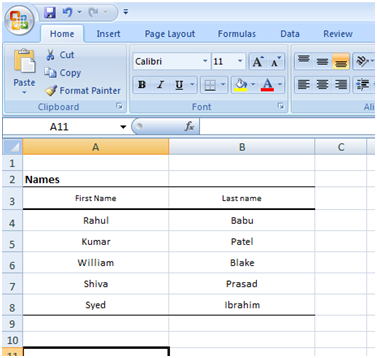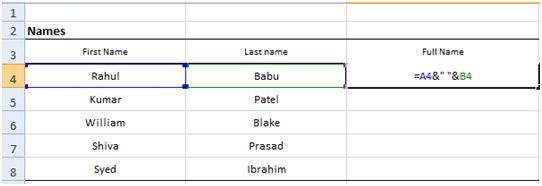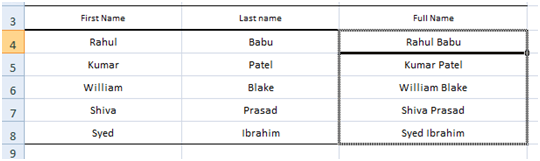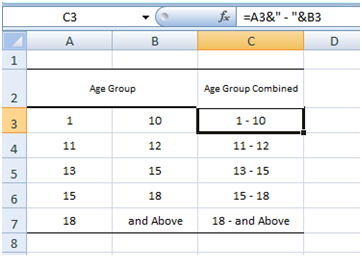# HOW TO – Combine Data in Two Cells into One

Though many Excel users are familiar with the operators(+,-,*,/ etc.) and built in functions(=Sum(A1:A4), Rand(), etc.) a few know a very useful yet insignificant ampersand (&) operator. This operator derived from the Excel’s Visual Basic base can get some tedious tasks in office a breeze.

Let’s, take the following example:In the above screenshot, names of five individuals split into first and last names are given. If one wants to combine these names into one cell then the first thing that comes to an excel user is the classic copy and paste. But this task can be quiet monotones if the list length is say hundred or thousand. In this situation “&” is what you need.

Type on to the cell where you want to combine the names, the below formulae:

= [Cell address with the first name(Here A4)] & “ [Space]” & [Cell address with the last name(Here B4)]Drag down the formulaeAnd your job is complete

You can use this technique for any kind of combining activities in excel, including numerals and strings.

Another is given below for reference: